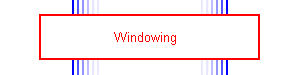# WindowingOnce the binaural signal is split into a number of frequency bands, rectified and filtered, it is then divided into short time windows in order to measure changes in the properties of the signal over time. The length of the window that is used in the measurement needed to match the temporal response of the relevant parts of the auditory system as accurately as possible, as well as take into account the fact that the window length should be at least five times the period of the lowest audio frequency of interest, in order to avoid end effects [Ifeachor and Jervis 1993].

## Optimum window length

The length of the window that is employed was based on reviewing previous research that investigated the perception of signals whose IACC varied over time and the limited temporal resolution in perceiving variations in interaural time and level differences. Research into the temporal resolution of IACC perception indicated that the optimum window length was between 35ms and 243ms, depending on the experiment and the subject. A mean value was found to be at approximately 85ms. Estimations based on the temporal resolution of the perception of stimuli with varying interaural time and level differences resulted in an optimum window length of approximately 50ms. This was discussed in [Mason et al 2003].

As a result of this research, the measurements made using the model have the option of using any window length. However, it is most common to use window lengths of between 35ms and 85ms, depending on whether the results are intended to predict the most critical levels or a more widely applicable value.

## Window overlap

If the audio signal was separated into non-overlapping windows, the results of the cross-correlation calculations would depend a great deal on position of the window boundaries in the audio signal. Therefore, it is important to overlap the measurement windows. On the other hand if the windows were implemented so that they started from consecutive samples, this would give a large overlap between the individual calculations, and a large number of calculations would be required. In order to increase the speed of the measurement and to remove unnecessary overlap in the calculations, a spacing between each of the consecutive measurements was introduced. The calculations had to be frequent enough to allow the detection of relatively rapid variations in the interaural cross-correlation coefficient, as Pollack estimated that variations up to a rate of approximately 500Hz can be detected [Pollack 1978].

As a compromise between the maximum rate of variation that is detectable and the duration of time that is required to process a measurement, a gap of 64 samples was chosen (which gives a measurement every 1.45ms at a sample rate of 44.1kHz). This gives a maximum quantifiable rate of variation of the cross-correlation of approximately 345Hz (assuming a sample rate of 44.1kHz).

We believe that the maximum rate of variation in interaural cross-correlation coefficient that can be perceived as a variation in the perceived source width is much lower than 500Hz, though further research is required to examine this. Therefore, it is possible that the overlap employed in the windowing can be reduced in future.

## Window shape

At the moment the window shape that is used in the measurement is a rectangular window. Whilst it is unlikely that this precisely mimics auditory perception, it is difficult to accurately judge the window shape that is most appropriate. In this case, the rectangular window is used until there is sufficient evidence regarding what type of window function would be more appropriate.